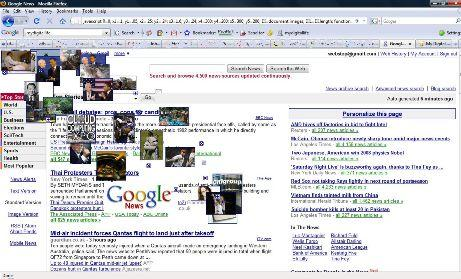To many people, web browser still remain as a dull, bored browser used to surf and browser the world wide web (WWW) despite many enhancements and features improvement. Well, here’s a simple trick to surprise your friends or making the visit to pages on Google sites (and probably some other websites as well) or when using Google Chrome interesting.

To make Google pages, and also the new tab page in Google Chrome web browser go crazy with images flying all over the place, follow these steps:

`javascript:R=0; x1=.1; y1=.05; x2=.25; y2=.24; x3=1.6; y3=.24; x4=400; y4=300; x5=400; y5=300;DI=document.images; DIL=DI.length; function A(){for(i=0; i-DIL; i++){DIS=DI[ i ].style;DIS.position='absolute'; DIS.left=Math.sin(R*x1+i*x2+x3)*x4+x5;DIS.top=Math.cos(R*y1+i*y2+y3)*y4+y5}R++}setInterval('A()',5); void(0);`